# 从零开始构建简单人工神经网络：1个输入层和1个输出层

### 为何从零开始?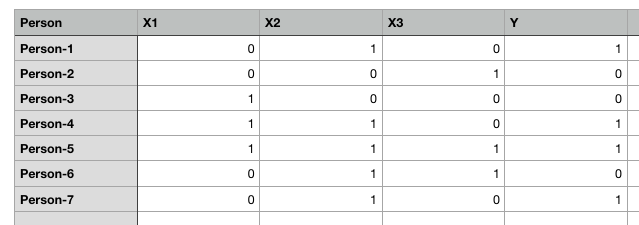ANN理论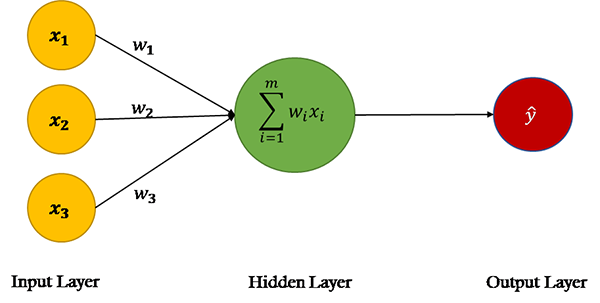X.W=x1.w1 + x2.w2 + x3.w3 + b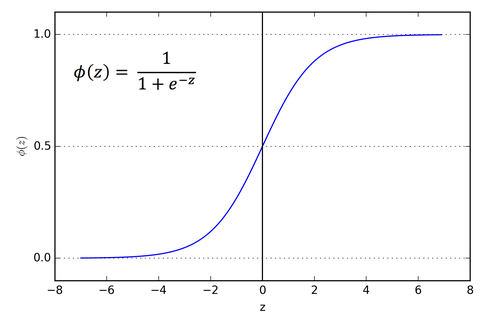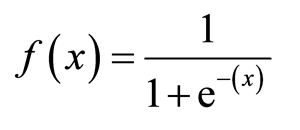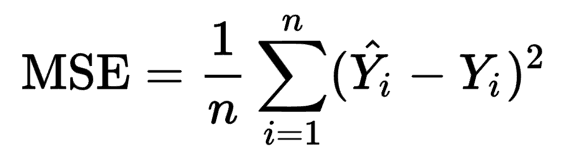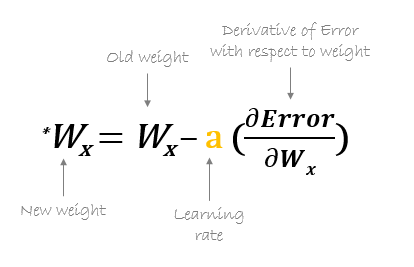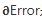是成本函数。上面的等式告诉我们找到关于每个权重和偏置的成本函数的偏导数，然后从现有权重中减去结果以得到新的权重。

### 使用numpy实现人工神经网络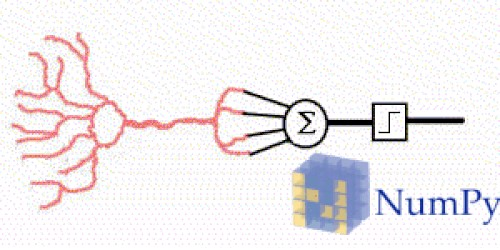1.定义自变量和因变量

2.定义超参数

3.定义激活函数及其导数

4.训练模型

5.做出预测

1. #Independent variables
2. input_set = np.array([[0,1,0],
3. [0,0,1],
4. [1,0,0],
5. [1,1,0],
6. [1,1,1],
7. [0,1,1],
8. [0,1,0]])#Dependent variable
9. labels = np.array([[1,
10. 0,
11. 0,
12. 1,
13. 1,
14. 0,
15. 1]])
16. labels = labels.reshape(7,1) #to convert labels to vector

1. np.random.seed(42)
2. weights = np.random.rand(3,1)
3. bias = np.random.rand(1)
4. lr = 0.05 #learning rate

1. def sigmoid(x):
2. return 1/(1+np.exp(-x))

1. def sigmoid_derivative(x):
2. return sigmoid(x)*(1-sigmoid(x))

1. for epoch in range(25000):
2. inputs = input_set
3. XW = np.dot(inputs, weights)+ bias
4. z = sigmoid(XW)
5. error = z - labels
6. print(error.sum())
7. dcost = error
8. dpred = sigmoid_derivative(z)
9. z_del = dcost * dpred
10. inputs = input_set.T
11. weights = weights - lr*np.dot(inputs, z_del)
12. for num in z_del:
13. bias = bias - lr*num

1. inputs = input_set

1. XW = np.dot(inputs, weights)+ bias

1. z = sigmoid(XW)

1. error = z - labels
2. print(error.sum())1. slope = input x dcost x dpred

1. dcost = error
2. dpred = sigmoid_derivative(z)
3. z_del = dcost * dpred
4. inputs = input_set.T
5. weights = weight-lr*np.dot(inputs, z_del)

1. for num in z_del:
2. bias = bias - lr*num

1. -0.001415035616137969
2. -0.0014150128584959256
3. -0.0014149901015685952
4. -0.0014149673453557714
5. -0.0014149445898578358
6. -0.00141492183507419
7. -0.0014148990810050437
8. -0.0014148763276499686
9. -0.0014148535750089977
10. -0.0014148308230825385
11. -0.0014148080718707524
12. -0.0014147853213728624
13. -0.0014147625715897338
14. -0.0014147398225201734
15. -0.0014147170741648386
16. -0.001414694326523502
17. -0.001414671579597255
18. -0.0014146488333842064
19. -0.0014146260878853782
20. -0.0014146033431002465
21. -0.001414580599029179
22. -0.0014145578556723406
23. -0.0014145351130293877
24. -0.0014145123710998
25. -0.0014144896298846701
26. -0.0014144668893831067
27. -0.001414444149595611
28. -0.0014144214105213174
29. -0.0014143986721605849
30. -0.0014143759345140276
31. -0.0014143531975805163
32. -0.001414330461361444
33. -0.0014143077258557749
34. -0.0014142849910631708
35. -0.00141426225698401
36. -0.0014142395236186895
37. -0.0014142167909661323
38. -0.001414194059027955
39. -0.001414171327803089
40. -0.001414148597290995
41. -0.0014141258674925626
42. -0.0014141031384067547
43. -0.0014140804100348098
44. -0.0014140576823759854
45. -0.0014140349554301636
46. -0.0014140122291978665
47. -0.001413989503678362
48. -0.001413966778871751
49. -0.001413944054778446
50. -0.0014139213313983257
51. -0.0014138986087308195
52. -0.0014138758867765552
53. -0.0014138531655347973
54. -0.001413830445006264
55. -0.0014138077251906606
56. -0.001413785006087985
57. -0.0014137622876977014
58. -0.0014137395700206355
59. -0.0014137168530558228
60. -0.0014136941368045382
61. -0.0014136714212651114
62. -0.0014136487064390219
63. -0.0014136259923249635
64. -0.001413603278923519
65. -0.0014135805662344007
66. -0.0014135578542581566
67. -0.0014135351429944293
68. -0.0014135124324428719
69. -0.0014134897226037203
70. -0.0014134670134771238
71. -0.0014134443050626295
72. -0.0014134215973605428
73. -0.0014133988903706311

1. single_pt = np.array([1,0,0])
2. result = sigmoid(np.dot(single_pt, weights) + bias)
3. print(result)

1. [0.01031463]

1. single_pt = np.array([0,1,0])
2. result = sigmoid(np.dot(single_pt, weights) + bias)
3. print(result)

1. [0.99440207]

### 结论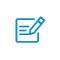扫码入群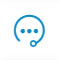咨询反馈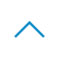返回顶部